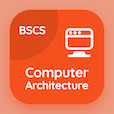Colleges Online Courses

College Math Quizzes

College Math Quiz PDF - Complete

# Double Angle Identities Multiple Choice Questions p. 137

Study Double Angle Identities multiple choice questions and answers, double angle identities quiz answers PDF 137 to study College Math course online. Double Angle Identities MCQ trivia questions, double angle identities Multiple Choice Questions (MCQ) for online college degrees. "Double Angle Identities MCQ" PDF eBook: period of trigonometric functions, nature of roots of quadratic equation, triple angle identities, trigonometric ratios of allied angles, double angle identities test prep for free online college courses.

"Cosα-sinα/cosα+sinα" MCQ PDF: sec2α-tan2α, sec2α+tan2α, csc2α-cot2α, and csc2α+cot2α for ACT subject test tutoring. Learn double angle identities questions and answers to improve problem solving skills to learn free online courses.

## Double Angle Identities Questions and Answers MCQs

MCQ: Cosα-sinα/cosα+sinα

sec2α+tan2α
sec2α-tan2α
csc2α-cot2α
csc2α+cot2α

MCQ: Tan(-α) =

−tanα
tanα
cotα
cotα

MCQ: Sin9α =

4cos³3α-3cos3α
3cos³3α-4cos3α
3sin3α-4sin³3α
4sin3α-3sin³3α

MCQ: The discriminant of quadratic equation ax² +bx +c = 0 is

b² + 4ac
4ac-b²
b² - 4ac
a² - 4bc

MCQ: The period of 3cot(x/3) is

π

### More Quizzes from College Math Course